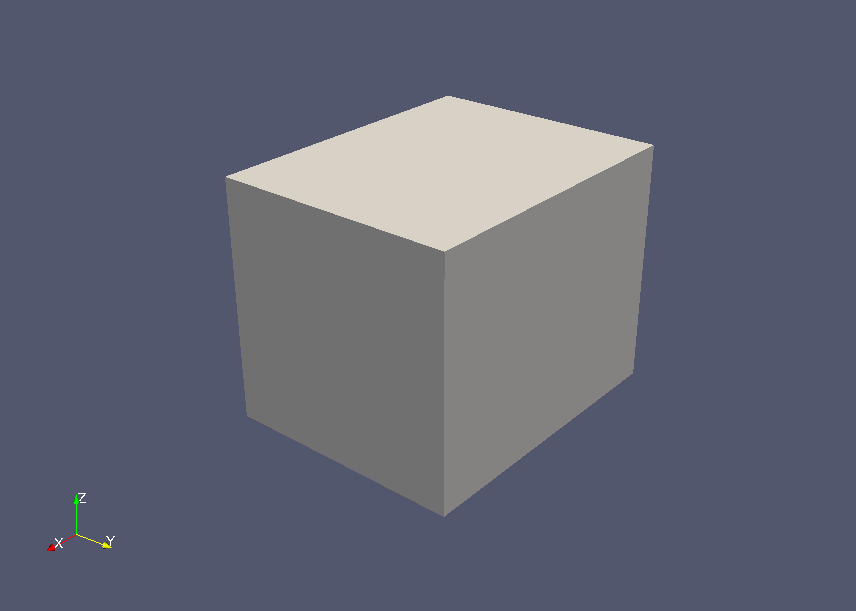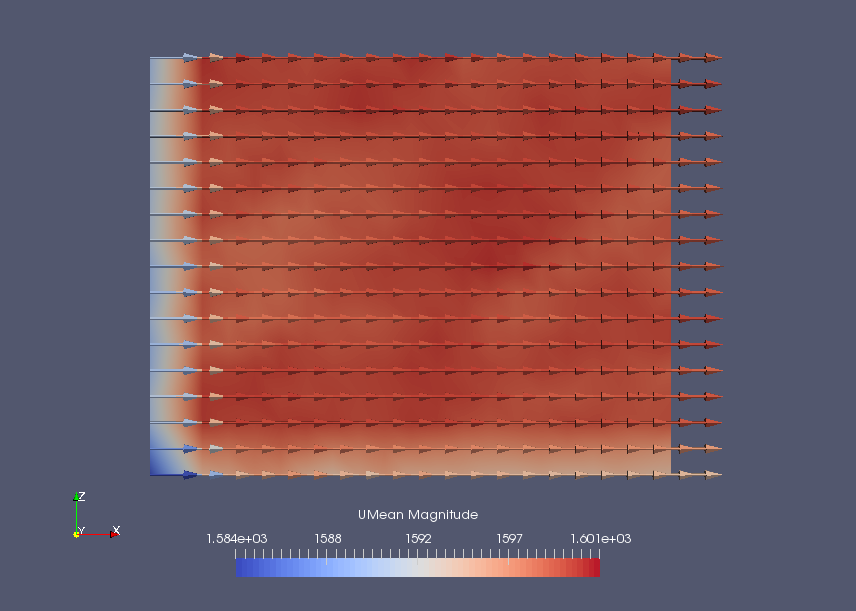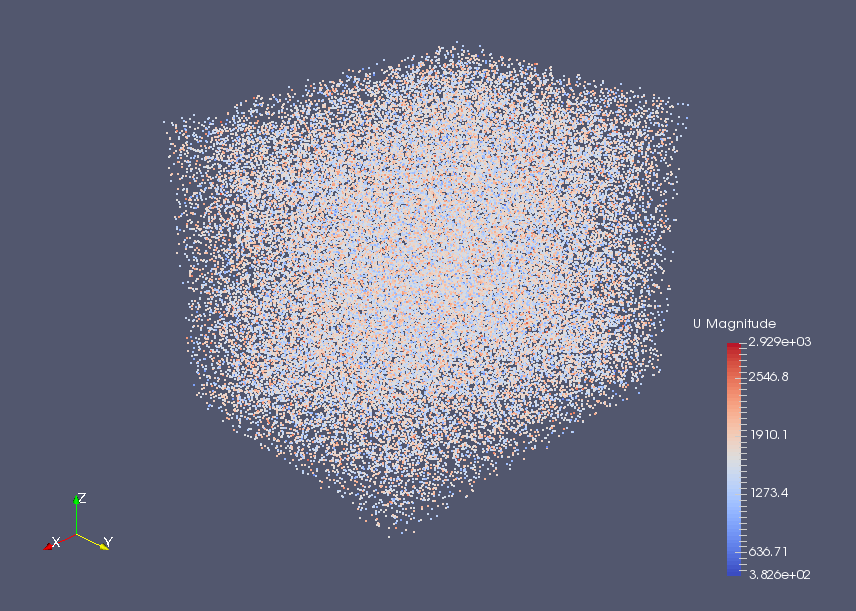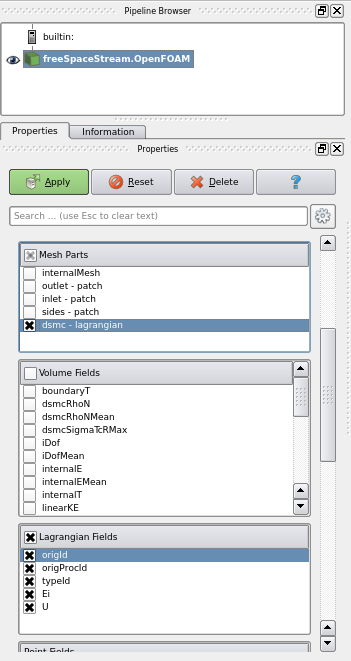﻿ Direct simulation Monte Carlo of oxygen/nitrogen mixtures (uniform flow) - XSim

# Direct simulation and Monte Carlo simulation of oxygen/nitrogen mixtures (uniform flow)

Update: January 3, 2018
OpenFOAM 4.x

## Case directory

\$FOAM_TUTORIALS/discreteMethods/dsmcFoam/freeSpaceStream

## Summary

We calculate the mixture gas flow of oxygen and nitrogen with the Direct Simulation Monte Carlo (DSMC) method. The Direct Simulation Monte Carlo (DSMC) method was proposed by G. A. Bird in the 1960s to calculate the flow of rarefied gases using the Boltzmann equation.

The properties of the gas are defined in the file "dsmcProperties" in the directory "constant", and the initial state is defined in the file "dsmcInitialiseDict" in the directory "system".

The size of the model in the X, Y, and Z axis directions is 10 cm, 8 cm, and 8 cm, respectively, and each face has a boundary condition of (1325, -352, 823) m/s flow velocity.Model geometry

Each variable in directory 0 is as follows.

• boundaryT - Boundary temperature
• boundaryU - Boundary velocity
• dsmcRhoN - DSMC particles Density
• fD - Force Density (stress vector)
• q - Wall heat flux
• iDof - Density of internal degrees of freedom
• internalE - Internal energy density
• linearKE - Linear kinetic energy density
• momentum - Momentum density
• rhoM - Mass density
• rhoN - Number density
• dsmcSigmaTcRMax - maximum value of "sigmaT × cR" in each cell (see Bird p220)

The calculation result is as follows.Mean velocity at the final timeDSMC particles at final time

## Visualization operations

To visualize DSMC particles, load the data into ParaView by the paraFoam command, and then set the following in the Properties tab of the pipeline browser and click the Apply button.

• Check only "dsmc" in the Mesh Parts list
• Uncheck all items in Volume Fields list
• Check all items in Lagrangian Fields listSelect display items

## Commands

cp -r \$FOAM_TUTORIALS/discreteMethods/dsmcFoam/freeSpaceStream freeSpaceStream
cd freeSpaceStream

blockMesh
dsmcInitialise
dsmcFoam

paraFoam

## Calculation time

31 minutes 40 seconds *Single, Inter(R) Core(TM) i7-2600 CPU @ 3.40GHz 3.40GHz